Time: 3 Hours                                                                                                     Max. Marks: 100

NOTE: There are 9 Questions in all.

·      Question 1 is compulsory and carries 20 marks. Answer to Q. 1. must be written in the space provided for it in the answer book supplied and nowhere else.

·      Out of the remaining EIGHT Questions answer any FIVE Questions. Each question carries 16 marks.

·      Any required data not explicitly given, may be suitably assumed and stated.

Q.1       Choose the correct or best alternative in the following:                                         (2x10)

a.       The output of a linear time-invariant system for a unit-step input is given by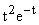.  The transfer function is given by

(A)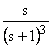(B)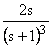(C)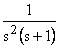(D)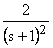b.      The Laplace transform of a unit-ramp function is

(A)    s                                                  (B)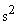(C)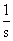(D)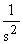c.   A plant with transfer function T(s) has output feedback with constant gain K applied to it.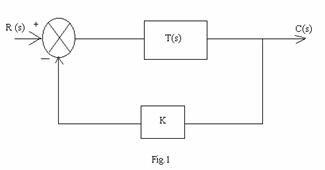The effect of the feedback is to

(A)    shift some zeros of T(s).                (B)  shift all zeros of T(s).

(C)  shift all poles and zeros of T(s)      (D)  shift only the poles of T(s)

d.   The characteristic equation of a closed-loop system is given by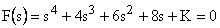.  If the system is to be stable, K must lie in the range

(A)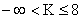(B)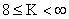(C)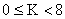(D)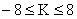e.   For a critically damped system, the following statement is not true:

(A)     A decrease in the damping will cause oscillatory behaviour.

(B)     The response to a unit step input converges asymptotically.

(C)     The system is marginally stable.

(D)    The step response never overshoots the final value.

f.    A second-order system has the transfer function: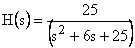.  The damped natural frequency of oscillation is

(A)     5                                                  (B)  4

(C)  3                                                  (D)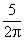g.   If a unit step input is applied to a system with transfer function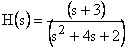, the steady-state output converges to

(A)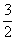(B)  0

(C)  1                                                  (D)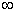h.   If the poles of the transfer function of a system are lying on the imaginary axis in s-plane, the system is

(A)   unstable                                        (B) stable

(C) marginally stable                            (D) conditionally stable

i.    The characteristic equation of a feedback-control system is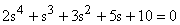.  The number of roots in the right half of s-plane are

(A)   2                                                   (B) 3

(C) 1                                                   (D) 0

j.    A unity feedback system has open-loop transfer function given by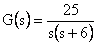.  The peak overshoot in the unit-step response of the system is approximately equal to

(A)  5%                                               (B)  20%

(C)  15%                                             (D) 10%

Answer any FIVE Questions out of EIGHT Questions.

Each question carries 16 marks.

Q.2           Consider the signal flow graph shown in Fig.2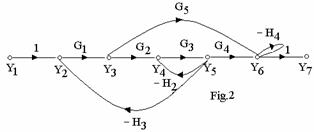Find

(i)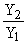(ii)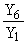(8+8)

Q.3     a.   Define the terms (i)gain margin and phase margin.  How will you find them from Nyquist plot?                                                                    (10)

b.   Explain in brief:

(i)                  Constant M-Circles

(ii)                Evaluation of closed-loop frequency response.                            (6)

Q.4     a.   Discuss the effects of P, P-I, P-D and P-I-D controllers on a second-order system.            (8)

b.   Explain in brief the effect of adding a pole or a zero in the left half of         s-plane in the open-loop transfer-function G(s) H(s) of a control system on the root-locus diagram.                                      (8)

Q.5           Consider the closed-loop system whose open-loop transfer function is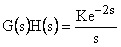.  Find the maximum value of K for which the system is stable.                                                            (16)

Q.6           Write notes on any TWO  of the following:-

(i)                  Uses of opamps for compensation.

(ii)                Tuning of PID controllers.

(iii)               Standard test signals.                                                             (8+8)

Q.7     a.   Give a network that provides lead compensation for a typical control system and explain its features.                                                                      (8)

b.   Distinguish between derivative error and derivative output compensations.           (8)

Q.8           A position control system with velocity feedback is shown in Fig.3.  What is the response C(t) to unit-step input?                                                  (16)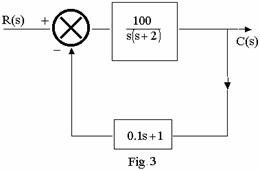Q.9     a.                                                              Sketch the root-loci for the system with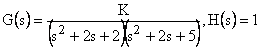.  Determine the exact points where the root-loci cross the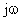-axis.                                                    (12)

b.   Explain the terms: Rise time, Peak time and Steady state error as defined for a second-order system.                                                                     (4)